# Test: Equations- 1

## 40 Questions MCQ Test Quantitative Aptitude for CA CPT | Test: Equations- 1

Description
Attempt Test: Equations- 1 | 40 questions in 40 minutes | Mock test for CA Foundation preparation | Free important questions MCQ to study Quantitative Aptitude for CA CPT for CA Foundation Exam | Download free PDF with solutions
QUESTION: 1

### Choose the most appropriate option (a) (b) (c) or (d) The equation –7x + 1 = 5–3x will be satisfied for x equal to:

Solution:

Take -7x to R.H.S. and 5 to L.H.S.,

Now on R.H.S., we have -3x+7x =4x and on L.H.S., we have -5+1= -4

So the equation becomes

-4 = 4x

=> x = -1

QUESTION: 2

### The Root of the equation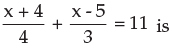Solution:

LCM of 4 and 3 = 12 , then 3(x+4) +4(x-5)=11 × 12 then 140/7 = 20 Answer

QUESTION: 3

### Pick up the correct value of x for X/30 =2/45

Solution:

If we cross multiply;

45x = 30*2
45x  =  60

x = 60/ 45

x  = 4/3

QUESTION: 4

The solution of the equation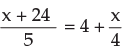Solution:

Taking the L.C.M. on R.H.S. as 4, and then on cross-multiplication, we get

4(x+24) = 5(16+x)

=> x = 16

QUESTION: 5

8 is the solution of the equation

Solution:

In option 2,

Took LCM .. SO u get 9x+36+2x+20=144.. so 11x= 88.. x=8

QUESTION: 6

The value of y that satisfies the equation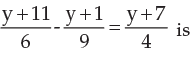Solution:
QUESTION: 7

The solution of the equation (p+2) (p–3) + (p+3) (p–4) = p(2p–5) is

Solution:

(p+2)(p-3)+(p+3)(p-4)=p(2p-5)

p^2-3p+2p-6+p^2-4p+3p-12=2p^2-5p

2p^2-2p-18=2p^2-5p

-2p+5p=18

3p=18

p=6

QUESTION: 8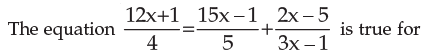Solution:
QUESTION: 9

Pick up the correct value x for which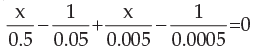Solution:

Put x=10
10/0.5-1/0.05+10/0.005-1/0.0005
= 20-20+2000-2000
=0

Hence, x=10 is the solution of the given equation.

QUESTION: 10

The sum of two numbers is 52 and their difference is 2. The numbers are

Solution:

Let the 2 nos. be x and x+2

Their sum is 52, so:

52 = x + (x+2)

52 = x + x + 2

50 = x + x

50 = 2x

25 = x

So your unknown numbers x and (x+2) are 25 and 27.

QUESTION: 11

The diagonal of a rectangle is 5 cm and one of at sides is 4 cm. Its area is

Solution:

Given :-

one side = a = 4cm

nd diagonal = h = 5cm

Let, the other side be 'b'

Now,

By applying pythgoras theorem ;

find base,

b^2 = h^2 - p^2

b^2 = (5)^2 - (4)^2

b^2 = 25 - 16

b^2 = 9

b = 3

So, the two sides are 4cm and 3cm

Then ;

Area of rect. = length * breadth

ar. = 4 * 3

ar. = 12 cm^2

QUESTION: 12

Divide 56 into two parts such that three times the first part exceeds one third of the second by 48. The parts are.

Solution:

Let the first part be x
other part is 56 - x
three times the first part exceeds one third of the second party by 48
3x = 1/3(56-x) + 48
9x = 56-x + 144
9x + x = 200
10x = 200
x = 20

First part, x =  20
Second part, 56 - x = 36

QUESTION: 13

The sum of the digits of a two digit number is 10. If 18 be subtracted from it, the digits in the resulting number will be equal. The number is

Solution:

x = 10's digit of a two digit number(Let)

y = units digit(Let)

n=Resulting equal digits(Let)

Condition 1-the sum of a two digit number is 10

x + y = 10

further simplifying

y = (10-x)

Condition 2-if 18 be subtracted from it the digits in the resulting number will be equal

10x + y - 18 = 10n + n

10x + y = 11n+ 18

Substitutituting values found above

10x + (10-x) = 11n + 18

10x - x = 11n + 18 - 10

9x = 11n+ 8

If we solve it we will found the values as - x = 7, y = 3, z = 5 and finally we can say the no. is 73.

QUESTION: 14

The fourth part of a number exceeds the sixth part by 4. The number is

Solution:

Let the number be 'x'.
By the problem,
(x/4)=(x/6)+4
⇒(x/4)-(x/6)=4
⇒(3x-2x)/12=4
⇒x/12=4
⇒x=4×12
⇒x=48.
Therefore, the number is 48.

Verification:
48/4=48/6+4
12=8+4
12=12
∴LHS=RHS.
So,the number is 48.

QUESTION: 15

Ten years ago the age of a father was four times of his son. Ten years hence the age of the father will be twice that of his son. The present ages of the father and the son are.

Solution:

F-10 = 4(S-10) on solving this one gets F - 4S = -30....1

F = 10 = 2(S+10) on solving this one gets F - 2S = 10....2

solve both the equation and you get F= 50 S = 20

QUESTION: 16

The product of two numbers is 3200 and the quotient when the larger number is divided by the smaller is 2.The numbers are

Solution:

Let two no is x and y X›y

X*y=3200

x/y=2

x=2y

2y*y=3200

2y^2=3200

y^2=1600

y=✓1600

y=40

then x=2*40

x=80

QUESTION: 17

The denominator of a fraction exceeds the numerator by 2. If 5 be added to the numerator the fraction increases by unity. The fraction is.

Solution:

Let numerator be: x

Let denominator be: x+2

fraction = x/(x+2)

(x+5)/(x+2) = x/(x+2) + 1

(x+5)/(x+2) - x/(x+2) = 1

(x+5 -x)/(x+2)= 1

5/(x+2) = 1

5 = x+2

5-2 = x

x = 3

The fraction is 3/5.

QUESTION: 18

Three persons Mr. Roy, Mr. Paul and Mr. Singh together have Rs. 51. Mr. Paul has Rs. 4 less than Mr. Roy and Mr. Singh has got Rs. 5 less than Mr. Roy. They have the money as.

Solution:

Mr. Roy has = x

Mr. Pual has = x-4

Mr. Singh has = x-5

Together they have = 51

Mr. Roy + Mr. Pual + Mr. Singh = 51

x+(x-4)+(x-5)=51

3x-9=51

3x=51+9

3x=60

divide by 3

3x/3=60/3

x=20

Mr. Roy has = x = 20

Mr. Pual has = x-4 = 20-4 = 16

Mr. Singh has = x-5 = 20-5= 15

20+16+15=51

51=51

QUESTION: 19

A number consists of two digits. The digits in the ten’s place is 3 times the digit in the unit’s place. If 54 is subtracted from the number the digits are reversed. The number is

Solution:

Let the digit at Tens place be x

The digit at unit place be y

The Number is 10x + y

ACCORDING TO THE QUESTION :-

x = 3y ... eq. 01

According to the second condition :

10x + y - 54 = 10y + x

=> 9x - 9y = 54

=> 9(x - y) = 9 × 6

=> x - y = 6 ... eq.02

Now we can solve the Equation 01 and 02 by substitution Method :-

x - y = 6

=> 3y - y = 6 [ from eq.01 , x = 3y ]

=> 2y = 6

=> y = 3

Now we can substitute the value of y in eq. 01 to get value of x

x = 3y

=> x = 3 × 3

=> x = 9

Hence the number is

Hence the number is 10x + y = 90 + 3 = 93 (ANS)

QUESTION: 20

One student is asked to divide a half of a number by 6 and other half by 4 and then to add the two quantities. Instead of doing so the student divides the given number by 5. If the answer is 4 short of the correct answer then the actual answer is

Solution: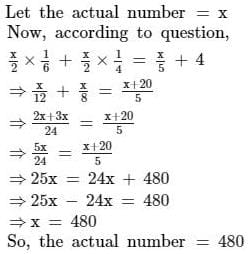QUESTION: 21

If a number of which the half is greater than 1/5th of the number by 15 then the number is

Solution:

Let it be x

x/2-x/5=15

3x/10=15

x/10=5

x=50

QUESTION: 22

The solution of the set of equations 3x + 4y = 7, 4x – y = 3 is

Solution:

Here 3x+4y=7.........(1)

4x-y=3........(2)

eq(1) * 1 --- 3x +4y =7
.
eq(2) * 4--- 16x -4y =12

................................................................
19x=19

=>x=19/19=1 ........

from eq(1) 3x + 4y=7

=>3*1 + 4y=7

=>4y = 7-3=4

=> y= 4/4=1 .

QUESTION: 23

The values of x and y satisfying the equations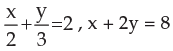are given by the pair.

Solution:
QUESTION: 24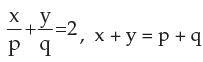are satisfied by the values given by the pair.

Solution:

According to the given question,

i ) x + y = p + q

x = p + q - y ---------( 1 )

ii ) x / p + y / q = 2

Multiply each term with pq we get

qx + py = 2pq ---------( 2 )

Put x value , from ( 1 ) in equation ( 2)

q( p + q - y) + py = 2pq

pq + q^2 - qy + py = 2pq

- qy + py = 2pq - pq - q^2

y ( - q + p) = pq - q^2

y ( p - q ) = q ( p - q )

y = q ( p - q )/ ( p - q )

y = q -----( 3 )

Put y = q in ( 1 )

x = p + q - q

x = p

Therefore ,

x = p and y = q

QUESTION: 25

The solution for the pair of equations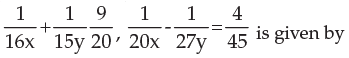Solution:

Put x=1/4 and y=1/3 in equation only option a is satisfied the equation LHS=RHS

QUESTION: 26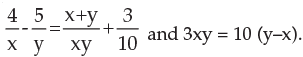The values of x and y are given by the pair.

Solution:

By option checking,

3xy = 10(y- x) so 3.2.5 =10(5-2)30=30

QUESTION: 27

The pair satisfying the equations x + 5y = 36,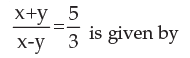Solution:
QUESTION: 28

Solve for x and y : x–3y =0, x+2y = 20. The values of x and y are given as

Solution:

given here

x-3y = 0...(1)

x+2y = 20...(2)

subtract equations (1) and (2)

we get ,

-5y = -20

=> y = (-20)/(-5)

=> y = 4

by (1).

x-3×4= 0

=> x= 12

Thus:-

Value of X = 12

Value of Y = 4

QUESTION: 29

The simultaneous equations 7x–3y = 31, 9x–5y = 41 have solutions given by

Solution:
QUESTION: 30

1.5x + 2.4 y = 1.8, 2.5(x+1) = 7y have solutions as

Solution:

I want to show you a good method to solve these equations.  Take time to read it and follow it.  This is the method in the International standards.  It is easy when you understand it.

1.5  x  + 2.4 y  -  1.8  =  0

2.5  x  -  7 y   + 2.5   =  0

For solving :  a x + b y + c  =  0     and    d x + e y + f  = 0 ,  we do as mentioned in the diagram enclosed.  This is the easier way.

-1.8      1.5      2.4      -1.8

2.5      2.5        -7       2.5

y                    x

x =   (-7*-1.8  -  2.4*2.5 ) / (2.5 * 2.4  - 1.5*-7)

= (12.6  -  6) / (6 + 10.5)  =  6.6/16.5 =  0.4  = 2/5

y =  (2.5*1.5 -  -1.8*2.5) / (2.5*2.4 - 1.5*-7)

= (3.75 + 4.5) / 16.5    =  8.25 / 16.5  = 0.5 = 1/2

QUESTION: 31

The values of x and Y satisfying the equations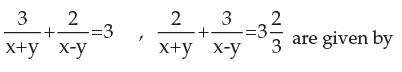Solution:

1/x+y=u and 1/x-y=v then

3u +2v = 3 x 2 = 6u + 4v = 6

2u + 3v = 11/3 x 3= 6u+ 9v =11subtracting we get

5v=5 --> v=1-->u=1/3

now 1/x+y = 1/3-->x+y=3

1/x-y = 1-->x-y=1 adding both we get 2x = 4--> x=2-->y=1 ANS)

QUESTION: 32

1.5x + 3.6y = 2.1, 2.5 (x+1) = 6y

Solution: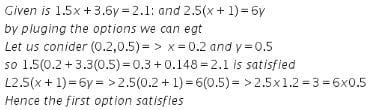QUESTION: 33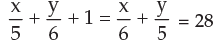Solution:

x/5 + y/6 = 27 ------( 1 )

x / 6 + y / 5 = 28 -----( 2 )

Multiply equations with 30 , we get

6x + 5y = 810 -----( 3 )

5x + 6y = 840 ----( 4 )

Multiply equation ( 3 ) with 6, we get

36x + 30y = 4860------( 5 )

Multiply equation with 5 , we get

25x + 30y = 4200 ------( 6 )

Subtract ( 6 ) from ( 5 ), we get

11x = 660

x = 660 / 11

x = 60

Put x = 60 in equation ( 5 ), we get

y = 90

Therefore,

x = 60 and y = 90

QUESTION: 34

If x/4 = y/3 = z/2 =k then what will be the value of x , y , z if 7x + 8y + 5z = 62

Solution:

Let x/4=y/3=z/2=k
x=4k
y=3k
z=2k
7x+8y+5z=62
⇒7*4k+8*3k+5*2k=62
⇒28k+24k+10k=62
⇒62k=62
⇒k=62/62
∴k=1

Hence,

x= 4

y=3

z= 2

QUESTION: 35

What will be the solution of these equations ax+by=a-b, bx-ay=a+b

Solution: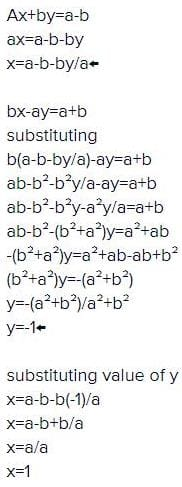QUESTION: 36

2x + 3y + 4z = 0, x + 2y – 5z = 0, 10x + 16y – 6z = 0

Solution:
QUESTION: 37

If x=a, y=b is the solution of the pair of equation x-y=2 and x+y=4 then what will be value of a and b

Solution:

X=a. y=b
a-b=2
a=2+b_(1)
a+b=4
2+b+b=4 _from (1)
2+2b=4
2b=2
b=1
a-1=2_(b=1)
a=3
ans:(3,1)

QUESTION: 38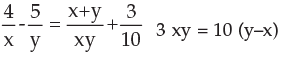Solution:

Solution :- 4/x - 5/y = (x+y)/ xy + 3/10

Taking L.C.M

(4y-5x)/ xy = (10x+10y+3xy) / 10xy

Cancelling both the 'xy's in the denominators

4y-5x = (10x+10y+3xy)/10

Cross multiplying, we get

40y-50x = 10x+10y+3xy

10x+10y+3xy-40y+50x=0

60x-30y+3xy=0....................... equation (1)

Equation (2)  3xy= 10(y-x)

3xy=10y-10x

-10x+10y-3xy = 0 .................. equation (2)

Solving both the following simplified equations

60x-30y+3xy =0...............(a)

-10x+10y -3xy = 0..............(b)

(a) + (b)

50x - 20y = 0

50x = 20y

x/y = 20/50

x : y :: 2:5

x=2 & y=5

QUESTION: 39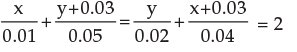Solution:

Put the value of x=0.01 and y=0.02 in equation
and solve it
option c satisfied the equation

QUESTION: 40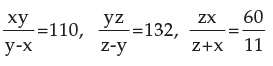Solution:

Put the value x=10, y=11and z=12
for example first equation is xy/y-x=110
put x=10and y=11
10*11/11-10
=110

We can solve this question by option checking
If you try to solve this question it will take too much time and you don't have enought time.Use Code STAYHOME200 and get INR 200 additional OFF Use Coupon Code